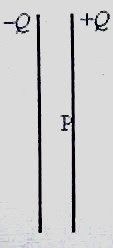## Pages

`“Life is like riding a bicycle.  To keep your balance you must keep moving.”–Albert Einstein`

## Tuesday, July 29, 2008

### AP Physics C – Electrostatics – Multiple Choice Practice Questions on Capacitors

Let us discuss some practice questions on capacitors meant for AP Physics C aspirants. You should try to find the answers yourself and then only go through the solution part. Here are the questions:(1) A parallel plate air capacitor with separation d between the plates negligibly small compared to the length and breadth of the plates, is fully charged by connecting it across a battery of emf V volt. If the capacitance of the capacitor is C and the area of each plate is A, the electric field at a point P in between the plates, at distance d/4 from the positive plate (Fig.) is

(a) CV/ε0A

(e) 3CV/0A

The point P being sufficiently away from the edge of the plate, the electric field at P is constant and is independent of the distance from the plate. The electric field due to a plane sheet of charge is σ/2ε0 where σ is the surface charge density (charge per unit area). [This can be easily obtained from Gauss’s law].

In the region between the plates, the fields due to the positive plate and the negative plate act in the same direction ( from positive plate to negative plate) and hence they add up to produce a net field of magnitude 2×σ/2ε0 = σ0.

Since σ = Q/A and Q = CV, the field at P is CV/ε0A.

[Note also that at a point outside, the electric field is zero since the positive and negative charges will produce equal and opposite fields].

(2) Two capacitors C1 and C2 are identical in all respects except for the dielectric media between their plates. C1 has air as dielectric where as C2 has a medium of dielectric constant K in place of air. The capacitor C1 is charged to V1 volt and the charging battery is disconnected. Then the uncharged capacitor C2 is connected across C1. If the common voltage across the capacitors is V2, the value of the dielectric constant K is

(a) V1/ V2

(b) V2/ V1

(c) (V1 + V2)/ (V1 V2)

(d) (V1 V2)/ V1

(e) (V1 V2)/ V2

If the capacitance of C1 is C the capacitance of C2 is KC.

Since the charge is conserved, the initial charge (Q) on C1 must be equal to the sum of the final charges on C1 and C2. Therefore we have

Q = CV1 = CV2 + KCV2

This gives K =(V1 V2)/ V2

(3) Two thin metal plates of the same area are given positive charges Q1 and Q2 and kept parallel to each other to form a capacitor of capacitance C. If Q2 > Q1 what will be the potential difference between the plates?

(a) (Q2 Q1) /2C

(b) (Q2 + Q1) /2C

(c) (Q2 + Q1) /C

(d) (Q2 Q1) /C

(e) (Q2 + Q1) /4C

The potential difference (V) between the plates is related to the electric field (E) between the plates and the distance (d) between the plates as

E = V/d

The plates produce opposing fields of magnitudes E1 (due to Q1) and E2 (due to Q2) in the region between the plates and the resultant field is directed from the plate carrying greater positive charge Q2 to that carrying smaller positive charge Q1.

Therefore, E = E2 E1 = V/d so that

V = (E2 E1)d

But E1 = σ1 /2ε0 and E2 = σ2 /2ε0 where σ1 and σ2 are the surface densities of charges on the plates given by σ1 = Q1/A and σ2 = Q2/A

Substituting these values, V = [(Q2/2ε0A) (Q1/2ε0A)] /d

Since ε0A/d = C, we obtain V = (Q2 Q1) /2C.

(4) A parallel plate capacitor with air as dielectric has plates of area A and separation d. It is charged to a potential difference V. The charging battery is then disconnected and the plates are pulled apart so that the separation becomes 3d. What is the work done for pulling the plates?

(a) ε0AV2/2d

(b) ε0AV2/3d

(c) ε0AV2/d

(d) 0AV2/d

(e) 2ε0AV2/3d

It is enough to find the difference between the energies of the capacitor. The potential difference between the plates will increase on pulling the plates apart; but the charge will remain unchanged. The energy (U1) before pulling is given by

U1 = Q2/2C where C is the initial value of capacitance given by C = ε0A/d.

When the separation between the plates is increased to 3d, the capacitance is reduced to C/3 and the energy (U2) is given by

U2 = Q2/(2C/3) = 3Q2/2C

The work done (W) for pulling the plates apart is given by

W = U2 U1 = 3Q2/2C Q2/2C = Q2/C

Since Q = CV where C = ε0A/d, we have

W = CV2 = ε0AV2/d

You will find some useful multiple choice questions with solution at physicsplus.